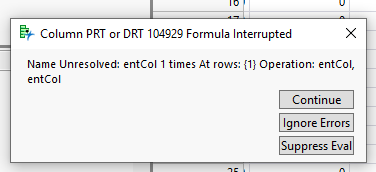Choose Language Hide Translation Bar
Highlighted

## Column Formulas Created by JSL Break After Sorting Data table

Hello,

I wrote a relatively simple script that would create a few columns with formulas to calculate some key values.

The script itself works fine, the columns get created and the values get populated.

The issue I am having is that if I then try to sort any column in the dt by either ascending or descending, those columns' created formulas will no longer produce anything. I can tell that the formulas do get reran, as I get an error stating that it does not recognize the name of the columns used in the formula:Here entCol is the variable for the column name I used in my script.

Is there a way to make sure that the column formulas that I script can be reran successfully if I choose to rearrange the data table?

Thanks,

Lain

2 ACCEPTED SOLUTIONS

Accepted Solutions
Highlighted

## Re: Column Formulas Created by JSL Break After Sorting Data table

Apparently there is something in the column formula that is order specific.  I the data in the formula is the way you want it, and you want the data to remain the same after sorting, you can specify to remove the formula, which will change the values in the column from calculated values, to static(hardcoded) values. Right click on the column header and select Column Info.  In the Column Info window, click on the Formula column property and then click on "Remove" button.

Jim
Highlighted

## Re: Column Formulas Created by JSL Break After Sorting Data table

It looks like you are saving the variable entCol in the formula instead of the column name itself.  As soon as the data table recalculates (not necessarily due to being sorted) the calculation fails.  Check out this link which shows a few methods of inserting the column name itself into the formula.

2 REPLIES 2
Highlighted

## Re: Column Formulas Created by JSL Break After Sorting Data table

Apparently there is something in the column formula that is order specific.  I the data in the formula is the way you want it, and you want the data to remain the same after sorting, you can specify to remove the formula, which will change the values in the column from calculated values, to static(hardcoded) values. Right click on the column header and select Column Info.  In the Column Info window, click on the Formula column property and then click on "Remove" button.

Jim
Highlighted

## Re: Column Formulas Created by JSL Break After Sorting Data table

It looks like you are saving the variable entCol in the formula instead of the column name itself.  As soon as the data table recalculates (not necessarily due to being sorted) the calculation fails.  Check out this link which shows a few methods of inserting the column name itself into the formula.

Article Labels